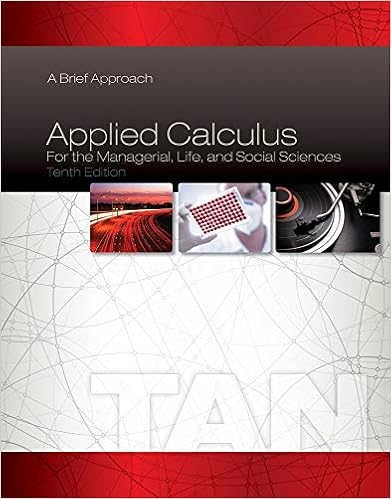# A higher saving rate leads to a a large capital stock

• Notes
• 22
• 100% (3) 3 out of 3 people found this document helpful

This preview shows page 20 - 22 out of 22 pages.

##### We have textbook solutions for you!
The document you are viewing contains questions related to this textbook.The document you are viewing contains questions related to this textbook.
Chapter 3 / Exercise 20
Applied Calculus for the Managerial, Life, and Social Sciences: A Brief Approach
TanExpert Verified
17) A higher saving rate leads to a:
18) The Golden Rule level of steady-state consumption per worker is:
19) If an economy is in a steady state with a saving rate below the Golden Rule level, efforts to increase the saving rate result in:
20) If Y=K^0.3L^0.7, then the per-worker production function is:
21) Which of the graphs illustrates the impact on the real exchange rate of contractionary fiscal at home, in the basic version of the small open economy model?
##### We have textbook solutions for you!
The document you are viewing contains questions related to this textbook.The document you are viewing contains questions related to this textbook.
Chapter 3 / Exercise 20
Applied Calculus for the Managerial, Life, and Social Sciences: A Brief Approach
TanExpert Verified
22) A country’s real exchange rate:
23) The efficiency of labor:
24) In the Solow model with technological progress, the steady-state growth rate of output per effective worker is:
25) In year one, capital stock was 6, labor input was 3, and output was 12. In year two, capital was 7, labor was 4, and output was 14. If shares of labor and capital were each ½, between the two years, total factor productivity:
26) According to the Solow model, persistently rising per-capita living standards can only be explained by:
27) In the Solow growth model, the steady-state growth rate of output per effective worker is ____, and the steady-state growth rate of output per worker is ____
28) In the Solow growth model with population growth and technological change, the break-even level of investment must cover:
29) In the Solow model with technological change, the Golden Rule level of capital is the steady state that maximizes:
30) The Solow residual equals the percentage change in output:
31) The Solow model with population growth but no technological change cannot explain persistent growth in standards of living because:
32) An appreciation of the real exchange rate in the basic model of a small open economy could be the result of:
33) One consequence of high inflation is a(n):
•••